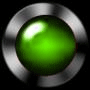RC time constants
predicting charge time

Capacitors charge and discharge at predictable rates similar to growth and decay curves in other areas of nature such as radioactivity or populations.

Lights that turn off automatically, timer circuits, oscillators that drive electronic instruments and wireless transmitters, all use the charging and discharging rates of RC circuits to determine periods of time.

A unit of time called a time constant can be calculated for and RC circuit that is a circuit with a resisitor and a capacitor in series.

The time constant is Tc = RC      where R = resistance C = capacitance

There are 5 time constants in the total time (T) to charge a capacitor through a resistor.

T = 5Tc

Example : calculate the time required to charge or discharge the following capacitor if the capacitor is

rated at 1000 uF and the resistor 6.7 Kohms.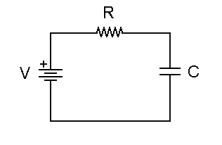R = 6700 (6.7Kohms)   C = 0.001F  (10-3 F = 1000uF)

Tc = RC

= 6700ohms x 0.001F = 6.7s (seconds)

T = 5Tc

= 5(6.7s) = 33.5s

Conclusions:  for T time to fully charge

AS R increases T increases  (limits rate of electron flow)

AS C increases T increases (takes longer to fill - size counts! -)

and the inverse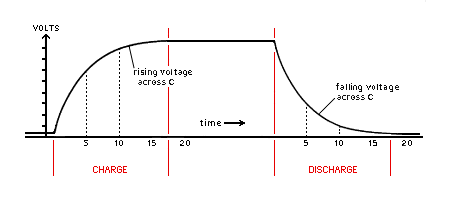Charge and Discharge Curves of a Capacitor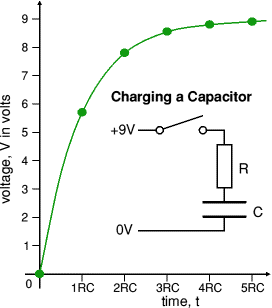note that the charge curve can be broken into 5 time constants the voltage across the capacitor increases at the fastest rate during the first time constant and the least in the last a capacitor never fully charges or discharges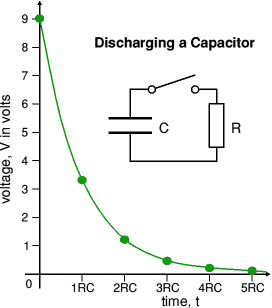note that the discharge curve can be broken into 5 time constants the voltage across the capacitor decreases at the fastest rate during the first time constant and the least in the last a capacitor never fully charges or discharges

The charge and discharge of RC circuits can trigger transistor switching oscillators.
Capacitor Charge/Discharge action animation
| 2 |

History of Capacitors     more on capacitors    other uses for a capacitor

more about capacitors in series and parallel

Capacitive Reactance a look ahead to your studies in AC theory   | 2 |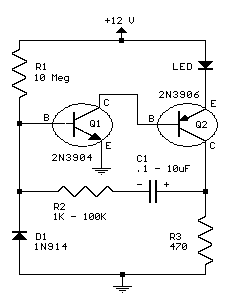LED FLASHER :    C1 the 10 uF cap and R2 the resistor control the flash rate
with the RC time